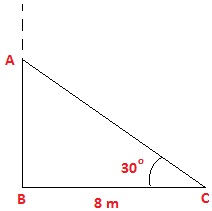# A tree breaks due to storm and the broken part bends so that the top of the tree touches the ground making an angle 30° with it. The distance between the foot of the tree to the point where the top touches the ground is 8 m. Find the height of the tree.

BC = 8 m To Find: Height of the tree, which is ABFrom diagram

Total height of the tree is the sum of AB and AC i.e. AB+AC

In rt ∆ABC,

BC = 8m

AB/BC = tan30°

AB/BC = 1/√3

AB = 8/√3 ——-(1)

Now,

BC/AC = cos30°

8/AC = √3/2

√3AC = 16

AC = 16/√3 ——(2)

From (1) and (2)

Height of tree = AB + AC

= 8/√3 * 16√3

= 8√3 m

8 * 1.73 = 13.84m

The height of the tree is 13.84(15)(4)